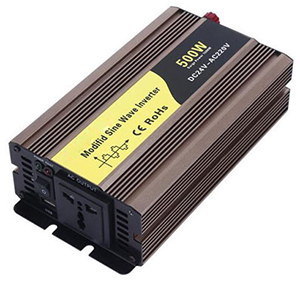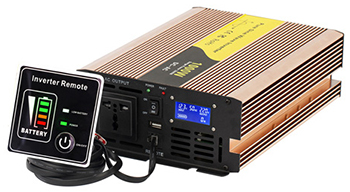# How to Select Power of Inverter for Home Use?

Power inverter has many specifications, and these specifications usually include rated power and peak power. The rated power is the continuous output power, which refers to the power that the inverter keeps working for a long time. The peak power is the starting power, which is generally twice the rated power. It is mainly used to meet the instantaneous peak value when individual home electrical appliances are started. For example, for a power inverter with 2000 watts continuous power, its peak power can reach 4000 watts. Therefore, for an inverter, its peak power must be able to meet the instantaneous power when the home appliance starts to ensure normal operation.

When selecting an inverter, its power should be higher than the starting power of the home electrical appliances used. When working, the inverter itself consumes part of the power. Its input power is greater than its output power. For example, if an inverter inputs 100 watts of direct current and outputs 85 watts of alternating current, its efficiency is 85%.

For example, the starting power of the motor is 1500 watts, and the peak power of the inverter is just 1500 watts. There will be efficiency loss during the conversion process, but the required power is actually not achieved. Therefore, when buying, a larger margin shall be left.

1. Heating wire, light bulb, and sun lamp and other purely resistive home appliances.For these home appliances, the power indicated on the electrical appliances is divided by 0.9, for example: 100W bulb, 100÷0.9=111. Therefore, with a 120W inverter, the bulb can be used safely.
2. TV set. There are two types of TVs, one is LCD TV and the other is CRT TV. For LCD TVs, as long as the inverter is twice the power of the TV standard. For example: LCD TV standard 100W, we can use it with a 300W power inverter. For CRT TVs, because of the large capacitance, the degaussing coil is equivalent to an inductive load, and the impact force is very strong, generally calculated as 10 times the peak value. For example: For 100W, peak 1000W, we need to be equipped with inverters above 500W to drive.
3. Computer. If it is a computer with a LCD, it should be selected by the standard power (computer host power) plus 90W. If it is a display of a picture tube, you need to calculate the peak power plus 90W according to the nominal power of the selected display (the host power is generally within 90W). If you feel it troublesome, just use a 500W power inverter, absolutely no problem.
4. Handheld power drill. Calculated by 3 times the peak value (drilling can only start after the no-load operation is stable, so the peak value is small). For example, for a 500W electric drill, peak value is 1500W, so select an 800W inverter. A 1500W electric drill is equipped with a 3000W inverter.
5. Pump. For home 500W water pump, it is calculated as 7 times (pumping water as soon as it starts, and it can only work at full load, and the peak value is large), and the peak value is 3500W, and 1800W inverter is required. A 750W pump must be equipped with a 2500W inverter to operate safely.
6. Air conditioning. Non-inverter air conditioners are calculated as 7 times the peak value, and inverter air conditioners are calculated as 4 times the peak value. For example, an air conditioner of 1000W per hour will be calculated by 7 times, which is 7000W. The peak value of a 3500W inverter is just 7000W. The inverter air conditioner is calculated by 4 times, the peak value of 1000W is 4000W, and the peak value of 2000W inverter is just 4000W. Therefore, a power inverter with 2000W continuous power is ideal for the inverter air conditioner.7. Car. Generally speaking, most passenger cars use 12V voltage, while trucks use 24V voltage. When selecting a car power inverter, please pay attention to buying an inverter suitable for the battery parameters. The battery voltage must be consistent with the nominal DC input voltage of the inverter. For example, a 24V inverter must correspond to a 24V battery.